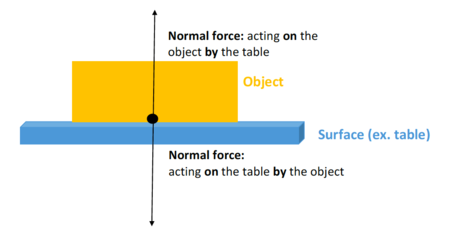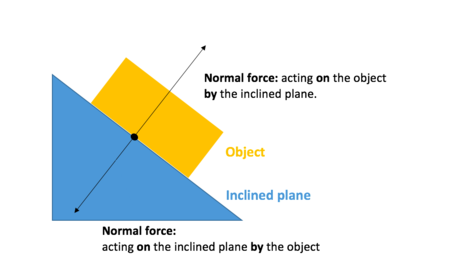# Normal forceFigure 1. The force arrow for either direction begins at the surface(the black dot). Due to Newton's third law, the normal forces will be equal in magnitude and in opposite direction. The normal force always makes a 90 degree angle with the surface (perpendicular).Figure 2. The normal forces that act on an object that is placed on an inclined plane. All the conditions in Figure 1 apply.

The normal force is an everyday force that is felt when a surface pushes against an object that is placed on that surface. For example, when a book is placed on a table, the normal force keeps the book from falling through the table. Gravity is pulling the book downward, but since the book isn't actually falling, something must be pushing it up. This force is called the normal force. The name 'normal' means perpendicular to the surface. Figure 1 and Figure 2 show how the the normal force acts on the object. Contrast to the gravitational force (whose force starts at the centre of the object)—the normal force begins at the surface.

The normal force arises from the electromagnetic force; specifically, the electrons in the book push against the electrons in the table. Since the electrons don't want to get any closer to each other, the book stays on top of the table.

The normal force is closely related to the friction force. Both are non-conservative forces, which can be seen when a ball bounces. The ball falls; gravity pulls it down, so it speeds up, while air drag somewhat slows the fall. When the ball hits the ground a huge normal force pushes upwards, making the ball bounce not quite as high as when it started. There is energy lost to changing the shape of the ball, which becomes thermal energy (warming the ball) and sound. The normal force can also be seen as non-conservative in the case of rolling resistance. The deformation of the tire (or wheel) means that energy is being lost to that change of shape.

See Florian Knorn's video below for an example of how the normal force deforms a tennis ball while bouncing: